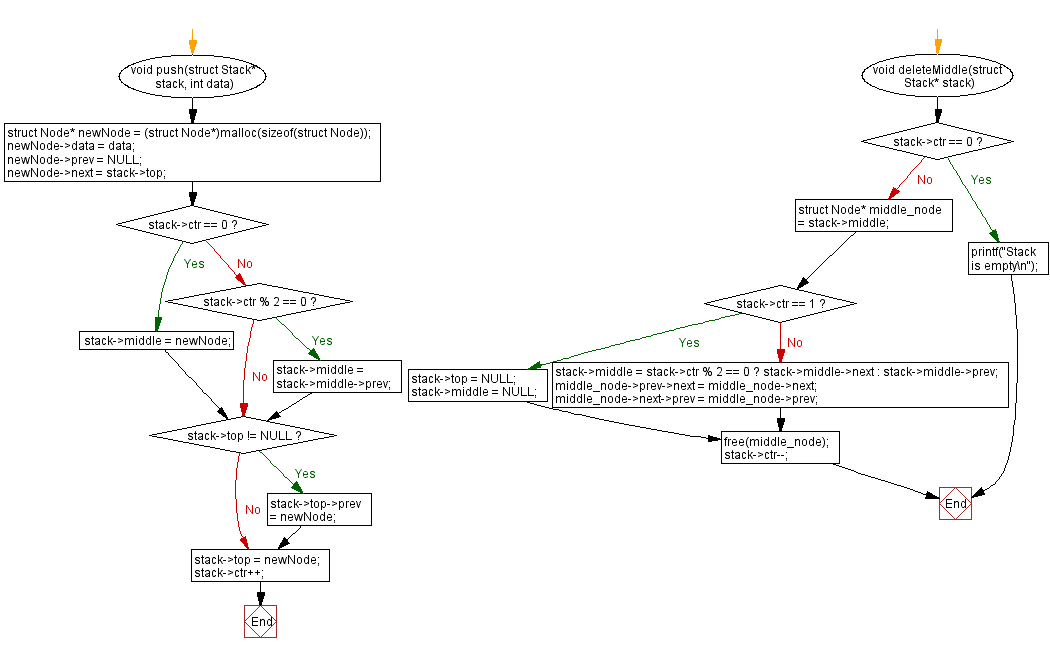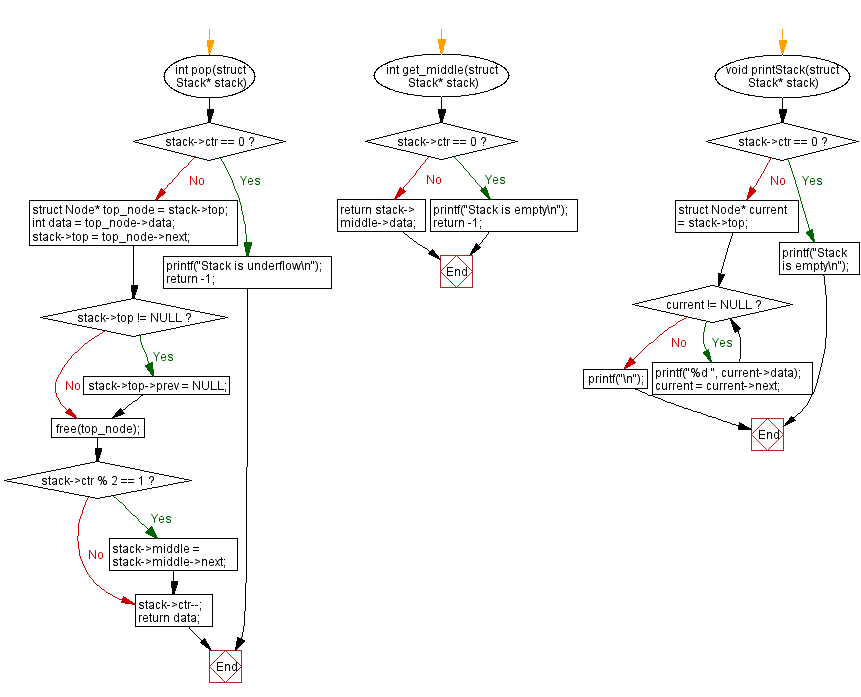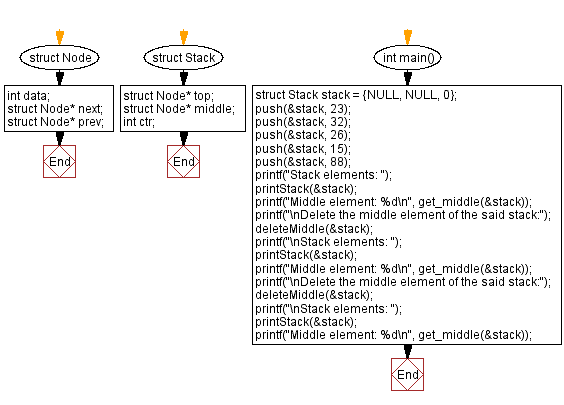﻿ C - Stack push, pop, get middle, and delete middle elements

# C Exercises: Stack push, pop, get middle, and delete middle elements

## C Stack: Exercise-13 with Solution

Write a C program to implement a stack that supports push, pop, get middle, and delete middle elements.

Sample Solution:

C Code:

``````#include <stdio.h>
#include <stdlib.h>

struct Node {
int data;
struct Node* next;
struct Node* prev;
};

struct Stack {
struct Node* top;
struct Node* middle;
int ctr;
};

void push(struct Stack* stack, int data) {
struct Node* newNode = (struct Node*)malloc(sizeof(struct Node));
newNode->data = data;
newNode->prev = NULL;
newNode->next = stack->top;

if (stack->ctr == 0) {
stack->middle = newNode;
} else if (stack->ctr % 2 == 0) {
stack->middle = stack->middle->prev;
}

if (stack->top != NULL) {
stack->top->prev = newNode;
}

stack->top = newNode;
stack->ctr++;
}

int pop(struct Stack* stack) {
if (stack->ctr == 0) {
printf("Stack is underflow\n");
return -1;
}

struct Node* top_node = stack->top;
int data = top_node->data;

stack->top = top_node->next;

if (stack->top != NULL) {
stack->top->prev = NULL;
}

free(top_node);

if (stack->ctr % 2 == 1) {
stack->middle = stack->middle->next;
}

stack->ctr--;

return data;
}

int get_middle(struct Stack* stack) {
if (stack->ctr == 0) {
printf("Stack is empty\n");
return -1;
}

return stack->middle->data;
}

void deleteMiddle(struct Stack* stack) {
if (stack->ctr == 0) {
printf("Stack is empty\n");
return;
}

struct Node* middle_node = stack->middle;

if (stack->ctr == 1) {
stack->top = NULL;
stack->middle = NULL;
} else {
stack->middle = stack->ctr % 2 == 0 ? stack->middle->next : stack->middle->prev;
middle_node->prev->next = middle_node->next;
middle_node->next->prev = middle_node->prev;
}

free(middle_node);
stack->ctr--;
}

void printStack(struct Stack* stack) {
if (stack->ctr == 0) {
printf("Stack is empty\n");
return;
}

struct Node* current = stack->top;
while (current != NULL) {
printf("%d ", current->data);
current = current->next;
}
printf("\n");
}

int main() {
struct Stack stack = {NULL, NULL, 0};
push(&stack, 23);
push(&stack, 32);
push(&stack, 26);
push(&stack, 15);
push(&stack, 88);
printf("Stack elements: ");
printStack(&stack);
printf("Middle element: %d\n", get_middle(&stack));
printf("\nDelete the middle element of the said stack:");
deleteMiddle(&stack);
printf("\nStack elements: ");
printStack(&stack);
printf("Middle element: %d\n", get_middle(&stack));
printf("\nDelete the middle element of the said stack:");
deleteMiddle(&stack);
printf("\nStack elements: ");
printStack(&stack);
printf("Middle element: %d\n", get_middle(&stack));
}
```
```

Sample Output:

```Stack elements: 88 15 26 32 23
Middle element: 26

Delete the middle element of the said stack:
Stack elements: 88 15 32 23
Middle element: 15

Delete the middle element of the said stack:
Stack elements: 88 32 23
Middle element: 32
```

Flowchart:C Programming Code Editor:

Have another way to solve this solution? Contribute your code (and comments) through Disqus.

What is the difficulty level of this exercise?

Test your Programming skills with w3resource's quiz.

﻿

## C Programming: Tips of the Day

C Programming - How do you pass a function as a parameter in C?

Declaration

A prototype for a function which takes a function parameter looks like the following:

```void func ( void (*f)(int) );
```

This states that the parameter f will be a pointer to a function which has a void return type and which takes a single int parameter. The following function (print) is an example of a function which could be passed to func as a parameter because it is the proper type:

```void print ( int x ) {
printf("%d\n", x);
}
```

Function Call

When calling a function with a function parameter, the value passed must be a pointer to a function. Use the function's name (without parentheses) for this:

```func(print);
```

would call func, passing the print function to it.

Function Body

As with any parameter, func can now use the parameter's name in the function body to access the value of the parameter. Let's say that func will apply the function it is passed to the numbers 0-4. Consider, first, what the loop would look like to call print directly:

```for ( int ctr = 0 ; ctr < 5 ; ctr++ ) {
print(ctr);
}
```

Since func's parameter declaration says that f is the name for a pointer to the desired function, we recall first that if f is a pointer then *f is the thing that f points to (i.e. the function print in this case). As a result, just replace every occurrence of print in the loop above with *f:

```void func ( void (*f)(int) ) {
for ( int ctr = 0 ; ctr < 5 ; ctr++ ) {
(*f)(ctr);
}
}
```

Ref : https://bit.ly/3skw9Um